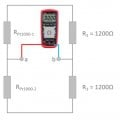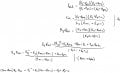# Resistance circuit problem.

#### EB(E/A)E

Joined Mar 29, 2020
24
Given the circuit below. Assume the PT1000 elements have same resistance. Lets name it Rpt. Find a expression for Rpt when you know R3 and the total resistance between point 'a' and point 'b' ---> Rab.My issue is that the problems I have encountered earlier has always included a voltage/amps source.
This circuit is made physically. I have measured the resistance between point 'a' and point 'b', and was given 1138 Ohms.
I'm aware the PT1000 elements have 1000 Ohms at 0 degrees Celcius, but that is kinda ignored in this problem I suppose.

How does this work when I measure between point a and b? Do I simply measure R3 and one PT1000 element? I dont think so.

My idea so far: I consider point 'a' a node point, node a. The same goes for point 'b', node b. Am I onto something here?

#### MrChips

Joined Oct 2, 2009
29,807
Do you know how to calculate equivalent resistance of resistors in series and in parallel?

•EB(E/A)E

#### ericgibbs

Joined Jan 29, 2010
18,213
hi EB,
If you redraw the circuit, now can you solve it.?
E

#### Attachments

•EB(E/A)E

#### EB(E/A)E

Joined Mar 29, 2020
24
Do you know how to calculate equivalent resistance of resistors in series and in parallel?
Yes, I believe I do so.
hi EB,
If you redraw the circuit, now can you solve it.?
E
That made it easier to see.
Im trying to make an expression of Rpt
I would assume its either an expression that goes like this:
(Rpt1 + Rpt2) = Something

or

Rpt1 * Rpt2 = Something

Ive tried this, im kinda stuck: See attachment#### ericgibbs

Joined Jan 29, 2010
18,213
hi Eb,
You are over thinking it.
What is the equivalent resistance of the left side network ie: 1k and 1k2 in series.??
The right side network will be same.
E

•EB(E/A)E

#### EB(E/A)E

Joined Mar 29, 2020
24
hi Eb,
You are over thinking it.
What is the equivalent resistance of the left side network ie: 1k and 1k2 in series.??
The right side network will be same.
E
It will be 1k + 1k 200 = 2200 Ohms
and right side will be the same, and in parallell it will be 2200 Ohms divided by 2 = 1100 Ohms

#### ericgibbs

Joined Jan 29, 2010
18,213

#### Attachments

•EB(E/A)E

#### EB(E/A)E

Joined Mar 29, 2020
24
hi EB,
Now that you now know the value, try building a simpler equation for solving a Wheatstone bridge equivalent resistance.
Sometimes just redrawing a circuit can you an insight into a simple method of solving.
E
Ive been unfortunately busy. I checked out the Wheatstone bridge circuit. In my case I would assume its balanced.

However, in my case I have two unknowns: Rpt1 and Rpt2.

I found this equation R1/R3 = R2/Rx

which equates in my circuit : Rpt1/Rpt2 = R3/R3

or have I lost it?

#### MrAl

Joined Jun 17, 2014
10,883
Keep in mind that measurements do not always match calculations simply because the measuring devices are not perfect and neither are real world components like resistors. Volt meters, current meters, Ohm meters, all have tolerances, and so do resistors, capacitors, and inductors. These tolerances cause errors in measurements.

•EB(E/A)E

#### MrAl

Joined Jun 17, 2014
10,883
I think I have it correct now, could anyone check it out?
see attached picture below, thank youView attachment 221743

I am not sure what you are doing here.

Why dont you first try to calculate the total resistance of just the top section of your circuit, just the 1000 ohm resistor and the 1200 ohm resistor, forget about the bottom two.
Can you calculate the resistance of those two?

•EB(E/A)E

#### EB(E/A)E

Joined Mar 29, 2020
24
I am not sure what you are doing here.

Why dont you first try to calculate the total resistance of just the top section of your circuit, just the 1000 ohm resistor and the 1200 ohm resistor, forget about the bottom two.
Can you calculate the resistance of those two?
Yes the Rpt is 1000 Ohms at 0 degrees celcius. Im trying to find a formula for Rpt so that I can calculate how much Ohms there is at Rpt when Rab is X amount.

PT1000 is temperature sensor.

#### ericgibbs

Joined Jan 29, 2010
18,213
hi EB.
You are showing two PT1000's in your circuit, is one at ambient and the other at a different temperature.?
If they are both a the same temperature the bridge will always be in balance at zero mV out.??

E

#### Attachments

•EB(E/A)E

#### EB(E/A)E

Joined Mar 29, 2020
24
@ericgibbs

they are presumely at same temperature ( and same resistance). I have measured Rab in a room at approx 20-21 C degrees. That gave me 1138 Ohms. By knowing that and what resistance R3 I have to find a formula for Rpt.

one of my classmates did this, Im not sure if its correct tho:
Code:
               (-2 * Rab * R3)
Rpt=       ----------------
( 2* Rab - 4*R3)
But I dont know how he got there. And Im unable to contact him.

Moderators note : used code tags to perserve spaces

Last edited by a moderator:

#### EB(E/A)E

Joined Mar 29, 2020
24

#### MrChips

Joined Oct 2, 2009
29,807
@MrChips
R12 = R1 + R2 = 2200 Ohms
Good. Now do the same for R34.

R12 and R34 are in parallel. What is the equivalent resistance (Req)?

As a final step, write out the equations in algebraic variables and solve for R1.

•EB(E/A)E